Home Practice
For learners and parents For teachers and schools
Textbooks
Full catalogue
Pricing SupportLog in

We think you are located in United States. Is this correct?

# 2.5 Metals, metalloids and non-metals

## 2.5 Metals, metalloids and non-metals (ESAAD)

The elements in the periodic table can also be divided according to whether they are metals, metalloids or non-metals. The zigzag line separates all the elements that are metals from those that are non-metals. Metals are found on the left of the line, and non-metals are those on the right. Along the line you find the metalloids. You should notice that there are more metals then non-metals. Metals, metalloids and non-metals all have their own specific properties.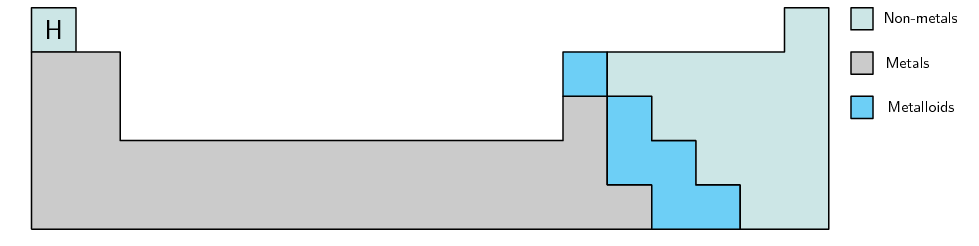Figure 2.6: A simplified diagram showing part of the periodic table.

### Metals (ESAAE)

Examples of metals include copper ($$\text{Cu}$$), zinc ($$\text{Zn}$$), gold ($$\text{Au}$$), silver ($$\text{Ag}$$), tin ($$\text{Sn}$$) and lead ($$\text{Pb}$$). The following are some of the properties of metals:

Copper wire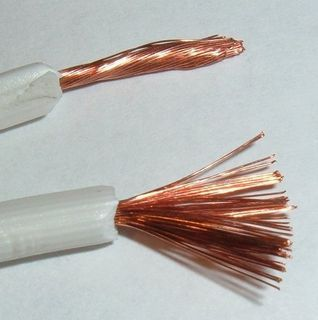• Thermal conductors

Metals are good conductors of heat and are therefore used in cooking utensils such as pots and pans.

• Electrical conductors

Metals are good conductors of electricity, and are therefore used in electrical conducting wires.

• Shiny metallic lustre

Metals have a characteristic shiny appearance and are often used to make jewellery.

• Malleable and ductile

This means that they can be bent into shape without breaking (malleable) and can be stretched into thin wires (ductile) such as copper.

• Melting point

Metals usually have a high melting point and can therefore be used to make cooking pots and other equipment that needs to become very hot, without being damaged.

• Density

Metals have a high density.

• Magnetic properties

Only three main metals (iron, cobalt and nickel) are magnetic, the others are non-magnetic.

You can see how the properties of metals make them very useful in certain applications.

## Looking at metals

1. Collect a number of metal items from your home or school. Some examples are listed below:

• hair clips

• safety pins

• cooking pots

• jewellery

• scissors

• cutlery (knives, forks, spoons)

2. In groups of $$\text{3}$$–$$\text{4}$$, combine your collection of metal objects.

3. What is the function of each of these objects?

4. Discuss why you think metal was used to make each object. You should consider the properties of metals when you answer this question.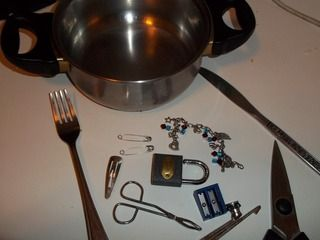### Non-metals (ESAAF)

In contrast to metals, non-metals are poor thermal conductors, good electrical insulators (meaning that they do not conduct electrical charge) and are neither malleable nor ductile. The non-metals include elements such as sulfur ($$\text{S}$$), phosphorus ($$\text{P}$$), nitrogen ($$\text{N}$$) and oxygen ($$\text{O}$$).

Sulfur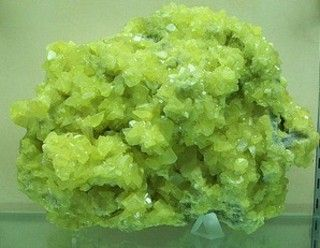### Metalloids (ESAAG)

Metalloids or semi-metals have mostly non-metallic properties. One of their distinguishing characteristics is that their conductivity increases as their temperature increases.

This is the opposite of what happens in metals. This property is known as semi-conductance and the materials are called semi-conductors. Semi-conductors are important in digital electronics, such as computers. The metalloids include elements such as silicon ($$\text{Si}$$) and germanium ($$\text{Ge}$$).

Silicon chips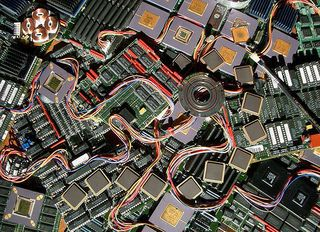temp text